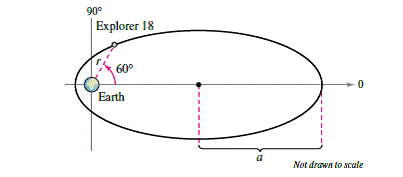Chapter 10.6, Problem 61E

Chapter
Section
Textbook Problem

# Explorer 18 On November 27, 1963, the United States launched Explorer 18. Its low and high points above the surface of Earth were approximately 119 miles and 123,000 miles (seefigure). The center of Earth is a focus of the orbit. Find the polar equation for the orbit and find the distance between the surface of Earth and the satellite when θ = 60 ∘ . (Assume that the radius of Earth is 4000 miles.)To determine

To Calculate:

The polar equation of the orbit of the satellite and the distance between the surface of earth and satellite when θ=600.

Explanation

Given:

The low and high points above the surface of earth, of the satellite explorer 18, which was launched by United States, were approximately 119 miles and 123,000 miles. Centre of the earth is the focus of its orbit and assume that radius of earth is 4000 miles. The provided figure is shown below:

Formula Used:

The polar equation of curve is given by r=ed1ecosθ.

Also, a=12(r0+r1) and c=(ar0).

Calculation:

Consider the provided figure shown below:

The low and high points above the surface of earth of the explorer 18, are approximately 119 miles and 123,000 miles and the radius of earth is 4000 miles. Therefore, the vertices are

(123000+4000,0)=(127000,0) and (119+4000,π)=(4119,π)

Let r0 be the distance from the focus of the satellite to the closest vertex and r1 be the distance to the farthest vertex,

Since r0=4119 and r1=127000. Thus, a=12(r0+r1)

a=12(127000+4119)=1311192=65559.5

And, c=(ar0)

c=(65559.54119)=61440.5

Now, the eccentricity is:

e=ca=61440.565559.5=0.937

Now, to calculate the value of d,

Consider the polar equation is r=ed1ecosθ

When θ=0r0=ed1ecos0=ed1e......(1)When θ=πr1=ed1ecosπ=ed1+e

### Still sussing out bartleby?

Check out a sample textbook solution.

See a sample solution

#### The Solution to Your Study Problems

Bartleby provides explanations to thousands of textbook problems written by our experts, many with advanced degrees!

Get Started

#### Find the limit. limr9r(r9)4

Single Variable Calculus: Early Transcendentals, Volume I

#### (rn)4r52n

Applied Calculus for the Managerial, Life, and Social Sciences: A Brief Approach

#### Simplify: 68

Elementary Technical Mathematics

#### Evaluate the integral. 38. /6/3sincotsecd

Single Variable Calculus: Early Transcendentals

#### In Exercises 724, compute the indicated products. 

Finite Mathematics for the Managerial, Life, and Social Sciences

#### The graph at the right has equation:

Study Guide for Stewart's Multivariable Calculus, 8th